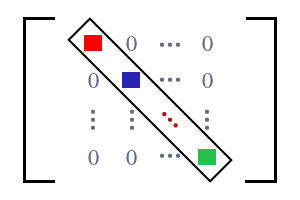# Diagonal Matrix

A square matrix whose non-diagonal elements are zero, is called a diagonal matrix.Diagonal matrix

Square matrices are appeared with zeros. In a special case, a square matrix contains zero as non-diagonal elements but it contains elements only on principal diagonal. Due to having elements on leading diagonal and having zeros as non-diagonal elements, the square matrix is recognized as a diagonal matrix.

$M=\left[\begin{array}{ccccc}{e}_{1⁣1}& 0& 0& \cdots & 0\\ 0& {e}_{2⁣2}& 0& \cdots & 0\\ 0& 0& {e}_{3⁣3}& \cdots & 0\\ ⋮& ⋮& ⋮& \ddots & ⋮\\ 0& 0& 0& \cdots & {e}_{m⁣m}\end{array}\right]$

The matrix is having elements ${e}_{1⁣1},{e}_{2⁣2},{e}_{3⁣3},\dots {e}_{m⁣m}$ only on principal diagonal but observe the elements on non-diagonal areas. All are zero elements at non-diagonal areas. Therefore, this type of matrix is called a diagonal matrix. The diagonal elements can be either equal or unequal elements.

It is simply expressed as $M=diag\left[\begin{array}{ccccc}{e}_{1⁣1,}& {e}_{2⁣2,}& {e}_{3⁣3,}& \cdots & {e}_{n⁣n}\end{array}\right]$

## Example

$D$ is a square matrix of order $5×5$. It is having $25$ element in five rows and five columns.

$D=\left[\begin{array}{ccccc}1& 0& 0& 0& 0\\ 0& –5& 0& 0& 0\\ 0& 0& 7& 0& 0\\ 0& 0& 0& 3& 0\\ 0& 0& 0& 0& 9\end{array}\right]$

The matrix $D$ is having two types of elements. One type of elements are nonzero elements and remaining all are zeros. Nonzero elements ($1,–5,7,3$ and $9$) are placed on the leading diagonal and remaining non-diagonal elements are zeros. Therefore, the matrix $D$ is known as a diagonal matrix.

The diagonal matrix $D$ is written in simple form $D=diag\left[\begin{array}{ccccc}1,& –5,& 7,& 3,& 9\end{array}\right]$

Email subscription
Math Doubts is a best place to learn mathematics and from basics to advanced scientific level for students, teachers and researchers. Know more
Follow us on Social Media
###### Math Problems

Learn how to solve easy to difficult mathematics problems of all topics in various methods with step by step process and also maths questions for practising.

Learn more